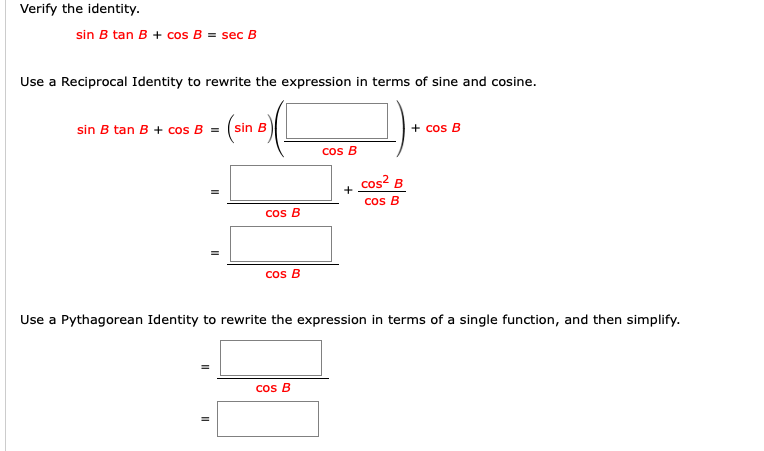# Verify the identity.sin B tan B + cos B = sec BUse a Reciprocal Identity to rewrite the expression in terms of sine and cosine.sin B tan B + cos B =sin B+ cos Bcos Bcos? BCos Bcos BCos BUse a Pythagorean Identity to rewrite the expression in terms of a single function, and then simplify.cos B

Question
8 viewshelp_outlineImage TranscriptioncloseVerify the identity. sin B tan B + cos B = sec B Use a Reciprocal Identity to rewrite the expression in terms of sine and cosine. sin B tan B + cos B = sin B + cos B cos B cos? B Cos B cos B Cos B Use a Pythagorean Identity to rewrite the expression in terms of a single function, and then simplify. cos B fullscreen
check_circle

Step 1

Given,

...

### Want to see the full answer?

See Solution

#### Want to see this answer and more?

Solutions are written by subject experts who are available 24/7. Questions are typically answered within 1 hour.*

See Solution
*Response times may vary by subject and question.
Tagged in

### Other